Next: Updating the Gates Up: CEM for Gaussian Mixture Previous: CEM for Gaussian Mixture

## Updating the Experts

To update the experts, we hold the gates fixed and merely take derivatives with respect to the expert parameters (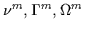or call them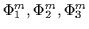). This derivative is simplified and set equal to zero as in Equation 7.17. This is our update equation for the experts. Note how each expert has been decoupled from the other experts, from the gates and from other distracting terms. The maximization reduces to the derivative of the logarithm of a single conditioned Gaussian. This can be done analytically with some matrix differential theory and, in fact, the computation resembles a conditioned Gaussian maximum-likelihood estimate in Equation 7.18 . The responsibilities for the assignments are the standard normalized joint responsibilities (as in EM). The update equation is effectively the maximum conditional likelihood solution for a conditioned multivariate Gaussian distribution.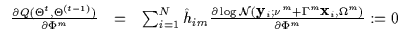(7.17)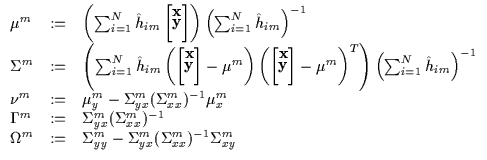(7.18)

We observe monotonic increases when the above maximization is iterated with the bounding step (i.e. estimation of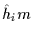). This result is not too surprising since we are effectively applying a version of EM to update the experts. At this point, we turn our attention to the still unoptimized gates and mixing proportions.Next: Updating the Gates Up: CEM for Gaussian Mixture Previous: CEM for Gaussian Mixture
Tony Jebara
1999-09-15